# 1576 Why Do the Digits of Every Clue in This Puzzle Add up to Nine?

### Today’s Puzzle:

The digits of each clue in this puzzle add up to nine. Relatively few Find the Factors puzzles can make that claim. Why can this one make it?

Can you write all the numbers from 1 to 10 so that this puzzle will function like a multiplication table?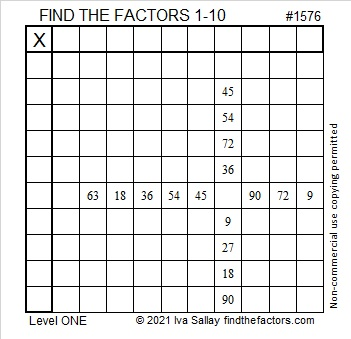### Factors of 1576:

1576 is divisible by a few powers of two, namely 1, 2, 4, and 8. Here is something for you to think about:

1576 is a whole number so it is divisible by 1.
6 is even so 1576 is divisible by 2.
7 is odd and 6 is not divisible by 4, so 1576 is divisible by 4.
5 is odd and 76 is not divisible by 8, so 1576 is divisible by 8.
1 is odd and 576 is divisible by 16, so 1576 is not divisible by 16.
(Each of those statements was also dependent on the statement before it.)

• 1576 is a composite number.
• Prime factorization: 1576 = 2 × 2 × 2 × 197, which can be written 1576 = 2³ × 197
• 1576 has at least one exponent greater than 1 in its prime factorization so √1576 can be simplified. Taking the factor pair from the factor pair table below with the largest square number factor, we get √1576 = (√4)(√394) = 2√394
• The exponents in the prime factorization are 3 and 1. Adding one to each exponent and multiplying we get (3 + 1)(1 + 1) = 4 × 2 = 8. Therefore 1576 has exactly 8 factors.
• The factors of 1576 are outlined with their factor pair partners in the graphic below.### More about the Number 1576:

1576 is the sum of two squares:
30² + 26² = 1576.

1576 is the hypotenuse of a Pythagorean triple:
224-1560-1576, calculated from 30² – 26², 2(30)(26), 30² + 26².
It is also 8 times (28-195-197).

1576 is also the difference of two squares in two different ways:
395² – 393² = 1576 and
199² – 195² = 1576.

# Sharing \$15.75 Worth of Puzzles

### Pattern Puzzle:

When I saw that 35 × 45 = 1575, I suspected a pattern. I made a chart to see if my suspicions were true, and they were! Can you look at the chart and tell me what that pattern is?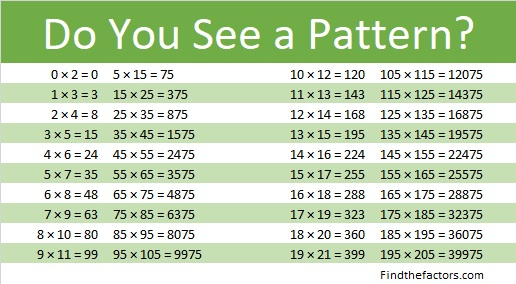If you were able to see that pattern, then look at each of these. They have patterns because the numbers in 3 × 17, 4 × 16, 5 × 15, 6 × 14, and 7 × 13 have a relationship. What is that relationship?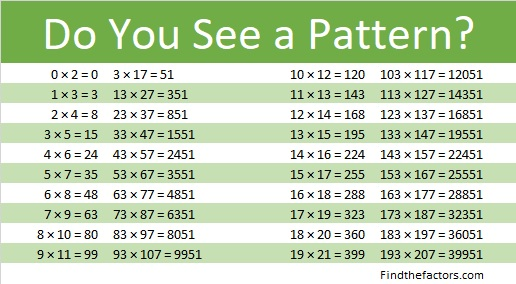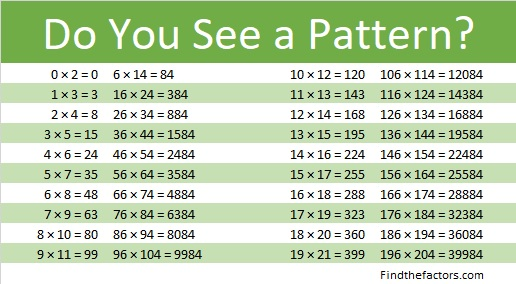### Factors of 1575:

We can use 35 × 45 = 1575 to make one of its many possible factor trees:• 1575 is a composite number.
• Prime factorization: 1575 = 3 × 3 × 5 × 5 × 7, which can be written 1575 = 3² × 5² × 7.
• 1575 has at least one exponent greater than 1 in its prime factorization so √1575 can be simplified. Taking the factor pair from the factor pair table below with the largest square number factor, we get √1575 = (√225)(√7) = 15√7.
• The exponents in the prime factorization are 2, 2, and 1. Adding one to each exponent and multiplying we get (2 + 1)(2 + 1)(1 + 1) = 3 × 3 × 2 = 18. Therefore 1575 has exactly 18 factors.
• The factors of 1575 are outlined with their factor pair partners in the graphic below.Can you use a different factor pair to create another factor tree for 1575? Will you always get 1575 = 3² × 5² × 7 in the end?

### Difference of Two Squares Puzzle:

1575 is the difference of two squares in NINE different ways:
788² – 787² = 1575,
264² – 261² = 1575,
160² – 155² = 1575,
116² – 109² = 1575,
92² – 83² = 1575,
60² – 45² = 1575,
48² – 27² = 1575,
44² – 19² = 1575, and
40² – 5² = 1575.

In money 1575¢ is represented as \$15.75. That’s the same as 63 quarters! Which of those differences of two squares is illustrated using quarters in the image below:Which of the nine difference of two squares above is illustrated in the following image?That image illustrates that \$15.75 is just one quarter away from the next perfect square dollar amount, \$16.00. Both 16 and 1600 are perfect squares. Can you make the rectangle below by moving just one row of quarters from the image above?8² – 1² = (8 – 1) × (8 + 1) = 63,
and might be the first step in understanding that  a² – b² = (a + b)(a – b) .

### Dividing Mixed Numbers Puzzles:

A quarter is 25¢. The reason a quarter is called a quarter is because it is a quarter or 1/4th of a dollar. We usually write dollar and cents together as decimals. A quarter is \$0.25.

Three quarters is 75¢ or \$0.75 and is 3/4ths of a dollar.

Two quarters is 50¢ or \$0.50 and is 2/4ths or one half of a dollar.

Representing 1575¢ in quarters can help you understand dividing mixed numbers like in the problem below:The answer to both questions is the same! Now try this one: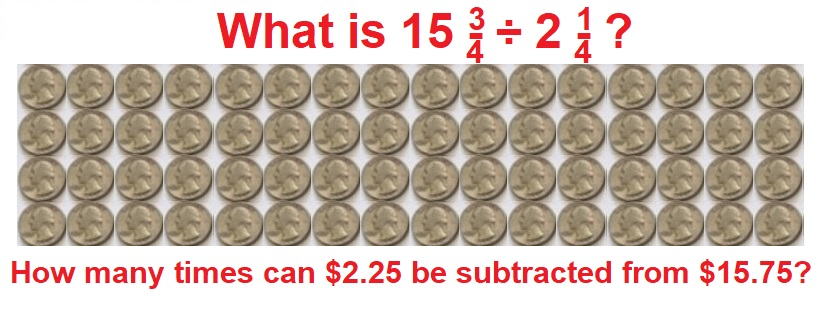You might not find this next example easy, but give it a look:Why is 13 in the denominator of the answer to both questions when it didn’t appear in either question? Where did the 13 come from?

Now try writing and solving your own problem: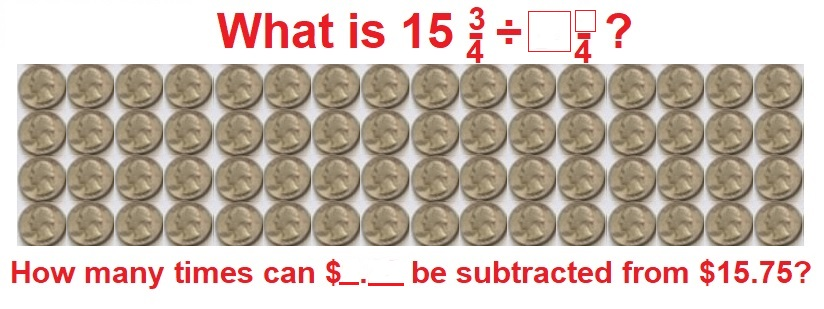Working with money often seems like more fun than working with numbers. I hope you enjoyed these puzzles today.

# 1574 Avoiding Fractions When Using the Substitution Method to Solve Simultaneous Equations

### The Substitution Method

Jo Morgan examines six methods of solving simultaneous linear equations in her wonderful book A Compendium of Mathematical Methods. Six is two more than the number of methods I had known previously. Jo loves pouring over vintage mathematics books to learn how concepts were taught in days gone by. She loves finding resources on Twitter to teach concepts and describes herself as a Resourceaholic. Today is her birthday, and this post is dedicated to her.

In my experience, the substitution method is often the first method taught to solve simultaneous linear equations, and usually, before the accompanying homework assignment is finished, fractions will be part of the solution process. Once students learn other methods, they usually abandon the substitution method with its troublesome fractions. However, the substitution method is really just as good as any other method, and fractions can actually be avoided when using it! I encourage students to use the substitution method and avoid fractions while they work.

In the table below I use the substitution method twelve ways to find one coordinate of the solution. (In each case the other coordinate could be found by substituting the known value back into one of the original equations and solving for the other coordinate.) Notice that only the first column uses fractions; the other two columns do not.Follow each solution process step by step. Do any of the methods seem less confusing than the others? How will you approach the problem the next time you are asked to solve simultaneous linear equations?

### Factors of 1574:

This is my 1574th post. Here is some information about that number:

• 1574 is a composite number.
• Prime factorization: 1574 = 2 × 787.
• 1574 has no exponents greater than 1 in its prime factorization, so √1574 cannot be simplified.
• The exponents in the prime factorization are 1 and 1. Adding one to each exponent and multiplying we get (1 + 1)(1 + 1) = 2 × 2 = 4. Therefore 1574 has exactly 4 factors.
• The factors of 1574 are outlined with their factor pair partners in the graphic below.### Another Fact about the Number 1574:

1574 is in just one Pythagorean triple:
1574-619368-619370, calculated from 2(787)(1), 787²-1², 787²+1².
It can also be calculated from 2(394²-393²), 4(394)(393), 2(394²+393²).

# 1573 Ring Out Wild Bells

### Today’s Puzzle:

Here are the first two verses of Alfred Lord Tennyson’s Ring Out Wild Bells:

Ring out, wild bells, to the wild sky,
The flying cloud, the frosty light;
The year is dying in the night;
Ring out, wild bells, and let him die.

Ring out the old, ring in the new,
Ring, happy bells, across the snow:
The year is going, let him go;
Ring out the false, ring in the true.

I think we are all ready for 2020 to “die” so we can ring in 2021. You can get started with this bell-shaped puzzle. Use logic to find its unique solution.Print the puzzles or type the solution in this excel file: 12 Factors 1558-1573

### Factors of 1573:

This is my 1573rd post, so let’s find the factors of 1573.

• 1573 is a composite number.
• Prime factorization: 1573 = 11 × 11 × 13, which can be written 1573 = 11² × 13
• 1573 has at least one exponent greater than 1 in its prime factorization so √1573 can be simplified. Taking the factor pair from the factor pair table below with the largest square number factor, we get √1573 = (√121)(√13) = 11√13
• The exponents in the prime factorization are 2 and 1. Adding one to each exponent and multiplying we get (2 + 1)(1 + 1) = 3 × 2 = 6. Therefore 1573 has exactly 6 factors.
• The factors of 1573 are outlined with their factor pair partners in the graphic below.### More about the number 1573:

1573 is the sum of two squares:
33² + 22² = 1573.

1573 is the hypotenuse of a Pythagorean triple:
605-1452-1573, calculated from 33² – 22², 2(33)(22), 33² + 22².
It is also (5-12-13) times 121.

# Factors and Facts for the Year 2021

### Countdown to 2021:

Here is a countdown you can use on New Year’s Eve to bring in 2021:make science GIFs like this at MakeaGif

### Math Facts about the Number 2021: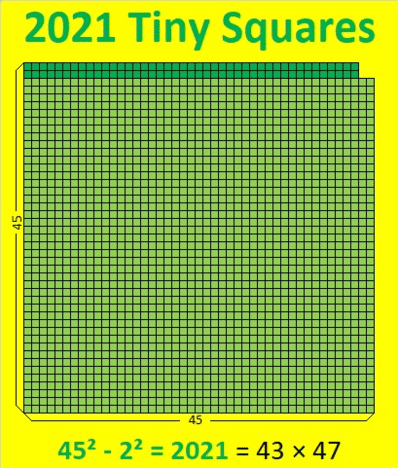make science GIFs like this at MakeaGif

2021 is the difference of two squares in two different ways:
45² – 2² = 2021 and
1011² – 1010² = 2021.

2021 is not the hypotenuse of any Pythagorean triples, but it is a leg in four:
180-2021-2029, calculated from 2(45)(2), 45² – 2², 45² + 2²,
2021-43428-43475, which is (43-924-925) times 47,
2021-47472-47515, which is 43 times (47-1104-1105)
2021-2042220-2042221, from 1011² – 1010², 2(1011)(1010), 1011² + 1010².2021 is one of the numbers in the table below because
2021² = 4084441, and their reverses are 1202² = 1444804.
For each pair of numbers in the table, the smaller number is listed first, but if you look carefully you will notice 2001, 2011, 2012, 2021, and 2022 in this century and 2101, 2102, 2111, and 2121 in the next.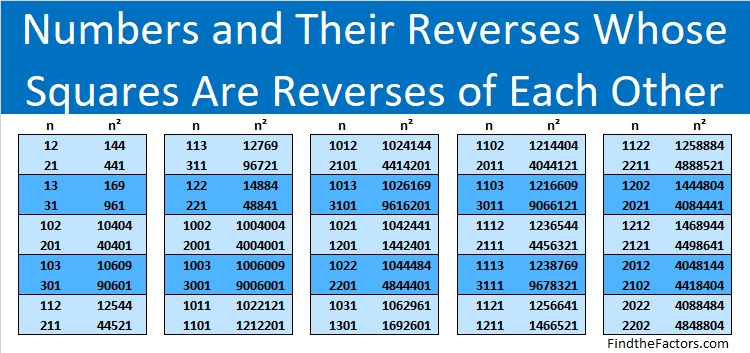### Factors of 2021:

The previous year had many negative factors, although it had just as many positive ones. I can predict that 2021 will have only four negative factors to go along with its four positive ones. I think we will see a lot of the negative factors at the beginning of the year, but hopefully, the positive ones will be more evident as the year goes on.

• 2021 is a composite number.
• Prime factorization: 2021 = 43 × 47.
• 2021 has no exponents greater than 1 in its prime factorization, so √2021 cannot be simplified.
• The exponents in the prime factorization are 1 and 1. Adding one to each exponent and multiplying we get (1 + 1)(1 + 1) = 2 × 2 = 4. Therefore 2021 has exactly 4 factors.
• The factors of 2021 are outlined with their factor pair partners in the graphic below.Here are tweets about the number 2021 in the order that I’ve seen them. If I see more, I’ll add more.

# 1572 Twelve Orientations of a Magic Snowflake

### Today’s Puzzle:

Without looking, can you decide where the numbers 1 to 13 go on a snowflake so that each of the six edges and each of the six lines of symmetry has the same sum? There is only one solution, but the snowflake can be put into twelve different orientations. Perhaps you solved that puzzle by writing in the numbers when I suggested it a few days ago. You will want to solve that puzzle first.

The twelve orientations are pictured below. They are all pictures of the same snowflake.Making a six-sided snowflake is more complicated than making a four-sided one. Rather than give step by step pictures of how I made the snowflake, I’ll just show one picture and explain the steps.1. Fold a piece of printer paper along a diagonal, cut off the excess to form a square.
2. Find the center of that diagonal and divide the folded paper into thirds that intersect the center. Cut the raw edge to make the largest possible equilateral triangle that is 6-sheets thick. Unfold. You now have a hexagon.
3. Fold an edge of the hexagon to the center but only make the crease go from one line of symmetry to the next one. Unfold. Repeat until all six edges have been folded to the center. This will create a medium-size hexagon inside the bigger hexagon.
4. Fold back into a 6-sheet-thick equilateral triangle. Divide the cut side into thirds and fold at a 90-degree angle to the cut side. Only crease from the edge to the medium-size hexagon. Unfold.
5. Cut along the fold you just made to create small slits in each of the twelve creases.
6. Cut a small slit along the folds of each side of the medium-size hexagon.
7. Fold back into a 6-sheet-thick equilateral triangle. Use decorative scissors to cut along the edge of the cut side of the triangle to make a pretty edge for the snowflake. Unfold until the only fold is along the diagonal.
8. Using a hole punch but only going halfway on the paper with the punch, make the center punch. It will unfold into a single punch. Fold back into a 6-sheet-thick equilateral triangle. Make a punch through all 6 sheets but keep the punch very close to the center punch. This will create the 7-punch small hexagon in the center of the snowflake.
9. Using the hole punch and any necessary creases, create hole punches representing the other numbers from 1 to 13. Take care that every edge and every line of symmetry has the same sum.

### Factors of 1572:

• 1572 is a composite number.
• Prime factorization: 1572 = 2 × 2 × 3 × 131, which can be written 1572 = 2² × 3 ×
• 1572 has at least one exponent greater than 1 in its prime factorization so √1572 can be simplified. Taking the factor pair from the factor pair table below with the largest square number factor, we get √1572 = (√4)(√393) = 2√
• The exponents in the prime factorization are 2, 1, and 1. Adding one to each exponent and multiplying we get (2 + 1)(1 + 1)(1 + 1) = 3 × 2 × 2 = 12. Therefore 1572 has exactly 12 factors.
• The factors of 1572 are outlined with their factor pair partners in the graphic below.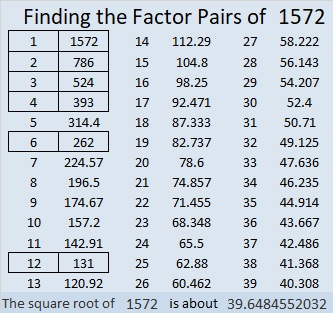### More about the Number 1572:

1572 is the difference of two squares two different ways:
394² – 392² = 1572 and
134² – 128² = 1572.

Since 1572 is divisible by 3, it is the magic sum of a magic hexagon. There isn’t room to punch enough holes in the hexagon, but you can see where all the numbers go below.# 1571 Candy Cane

### Today’s Puzzle:

The shepherds’ crooks from that first Christmas night have become the sweet candy canes we often see on today’s Christmas trees. Can you find the factors from 1 to 12 that will make this mystery level puzzle function like a multiplication table? Remember to use logic to find the factors.### Factors of 1571:

• 1571 is a prime number.
• Prime factorization: 1571 is prime.
• 1571 has no exponents greater than 1 in its prime factorization, so √1571 cannot be simplified.
• The exponent in the prime factorization is 1. Adding one to that exponent we get (1 + 1) = 2. Therefore 1571 has exactly 2 factors.
• The factors of 1571 are outlined with their factor pair partners in the graphic below.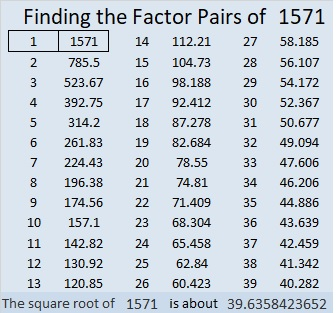How do we know that 1571 is a prime number? If 1571 were not a prime number, then it would be divisible by at least one prime number less than or equal to √1571. Since 1571 cannot be divided evenly by 2, 3, 5, 7, 11, 13, 17, 19, 23, 29, 31, or 37, we know that 1571 is a prime number.

### More about the Number 1571:

1571 is the sum of two consecutive numbers:
785 + 786 = 1571.

1571 is also the difference of two squares:
786² – 785² = 1571.

Do you see the relationship between those two facts?

# 1570 How to Solve the Dollar Tree Ball Puzzle

### Today’s Puzzle:

Perhaps you or one of your children will find the Dollar Tree Ball Puzzle in your stocking this year. Such was the case in my family.

A little bit of tugging here and there will help you pull apart this Dollar Tree puzzle ball, but how do you get it back together? After several failed attempts, you might turn to the internet for help. There are several videos showing how to put it back together, but they can be difficult to follow. The colors of the puzzle pieces might not match your ball. Thus, instructions on what to do with the yellow or green piece are not as helpful. People on the videos keep turning the ball so that makes the differences in color even more confusing and requires the videos to be played back over and over again.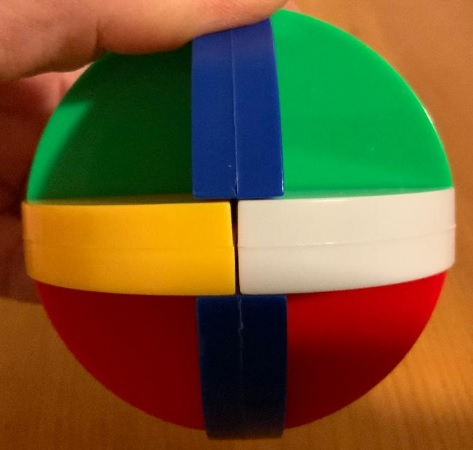Instead of posting yet another video, I decided to post still shots of each step with the Dollar Tree Puzzle Ball maintaining . The colors of your ball may not match the colors here, so match the indentations on your puzzle pieces instead of the colors. Turn your puzzle pieces so the indentations are in the same position as the indentations in the photos.

First 2 pieces: Find the two pieces shaped like these:Connect them so that together they look like an angel.  Third piece: There are actually two puzzle pieces that are shaped the same. Select one of them.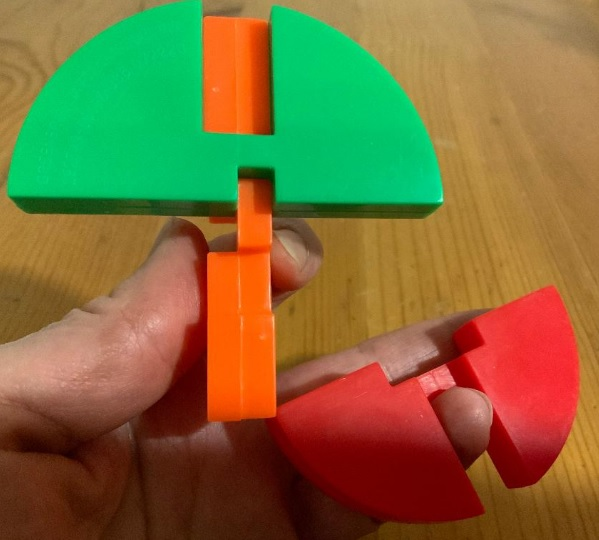Put your selected piece below the angel wings on the puzzle. Fourth piece: select the next piece that is shaped like the blue one below. See how the notch will fit over the center of the angel wings perfectly?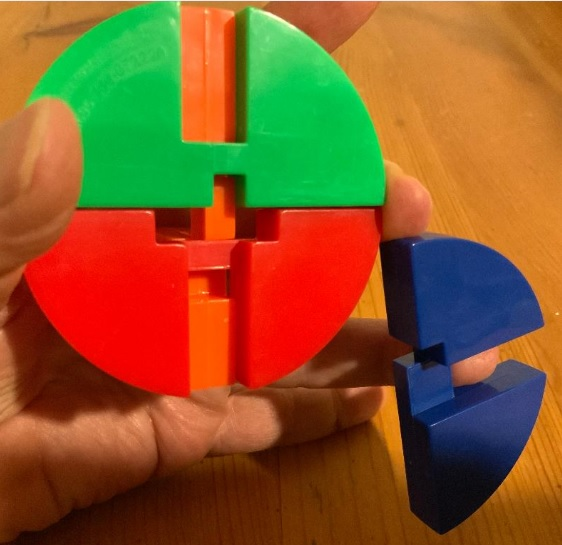Attach it to the puzzle as shown. Notice the two holes in the middle of the puzzle. The one on the left is a rectangle. The one on the right is a square.Fifth piece: Put the next piece in on the rectangle side with the solid part down. Push it in all the way to the middle.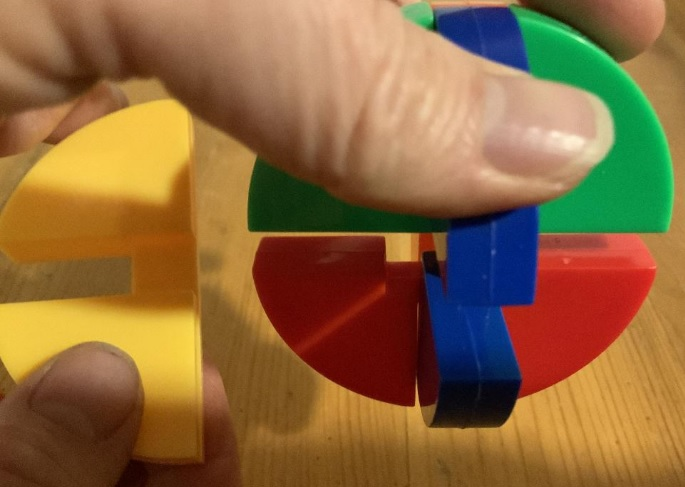Sixth piece: Put the last piece in. This is the piece that was identical to the third piece you put in the puzzle.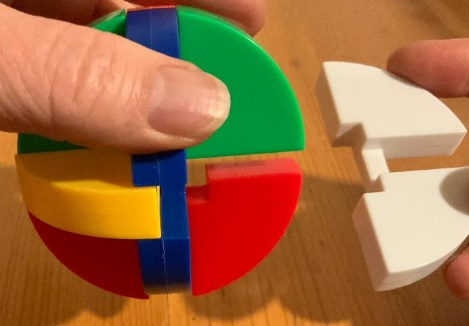Push it in all the way to the middle.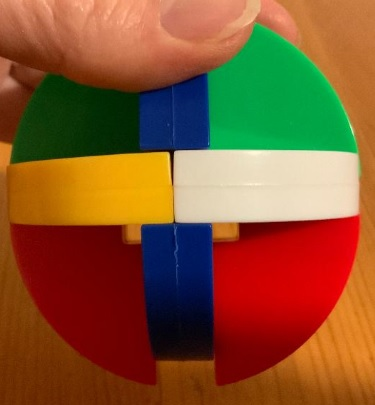Finally, push the bottom piece up to lock the puzzle into place.### Factors of 1570:

This is my 1570th post. Separating the number 1570 into puzzle pieces might seem difficult, but it only has three prime puzzle pieces: 2, 5, and 157. That last one might seem like it should be broken into more pieces, but it can’t be. Putting the puzzle pieces back together is much easier. Just multiply them all together to get 1570.

• 1570 is a composite number.
• Prime factorization: 1570 = 2 × 5 × 157.
• 1570 has no exponents greater than 1 in its prime factorization, so √1570 cannot be simplified.
• The exponents in the prime factorization are 1, 1, and 1. Adding one to each exponent and multiplying we get (1 + 1)(1 + 1)(1 + 1) = 2 × 2 × 2 = 8. Therefore 1570 has exactly 8 factors.
• The factors of 1570 are outlined with their factor pair partners in the graphic below.### More about the Number 1570:

1570 is the sum of two squares in two different ways:
29² + 27² = 1570, and
39² + 7² = 1570.

1570 is the hypotenuse of four Pythagorean triples:
112-1566-1570, calculated from 29² – 27², 2(29)(27), 29² + 27²,
546-1472-1570, calculated from 2(39)(7), 39² – 7², 39² + 7²
850-1320-1570, which is 10 times (85-132-157), and
942-1256-1570, which is (3-4-5) times 314.

# 1569 A Magic Hexagon Puzzle

### Today’s Puzzle:

The hexagon below has six edges and six lines going through its center. Can you write the numbers from 1 to 13 in the boxes so that the sums of the numbers along each of those lines are equal? The solution can be written 12 different ways, but they are all rotations and/or reflections of each other.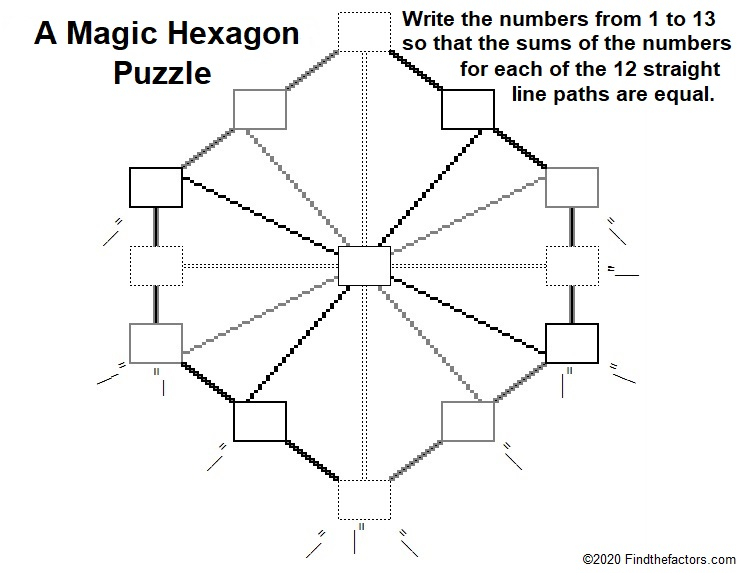It should be helpful to realize that
1 + 13 = 14,
2 + 12 = 14,
3 + 11 = 14,
4 + 10 = 14,
5 + 9 = 14, and
6 + 8 = 14.
That leaves a 7 without a partner. Where do you think the 7 should go? What do you think the magic sum of each of the twelve paths will be?

Should even numbers or odd numbers go in the corners of the hexagon?

### Factors of 1569:

This is my 1569th post. What are the factors of 1569?

• 1569 is a composite number.
• Prime factorization: 1569 = 3 × 523.
• 1569 has no exponents greater than 1 in its prime factorization, so √1569 cannot be simplified.
• The exponents in the prime factorization are 1 and 1. Adding one to each exponent and multiplying we get (1 + 1)(1 + 1) = 2 × 2 = 4. Therefore 1569 has exactly 4 factors.
• The factors of 1569 are outlined with their factor pair partners in the graphic below.### More about the Number 1569:

1569 is the difference of two squares:
263² – 260².

1569 is also the sum of the six numbers from 259 to 264.

Do you see any relationship between those two facts?

Since 1569 is divisible by 3, it is the magic sum of the magic hexagon shown below:# 1568 A Challenging Christmas Tree

### Today’s Puzzle:

Can you write the numbers from 1 to 10 in each of the boldly outlined columns and rows so that each quadrant of this Christmas tree puzzle behaves like a multiplication table? Remember to use logic and not guessing and checking.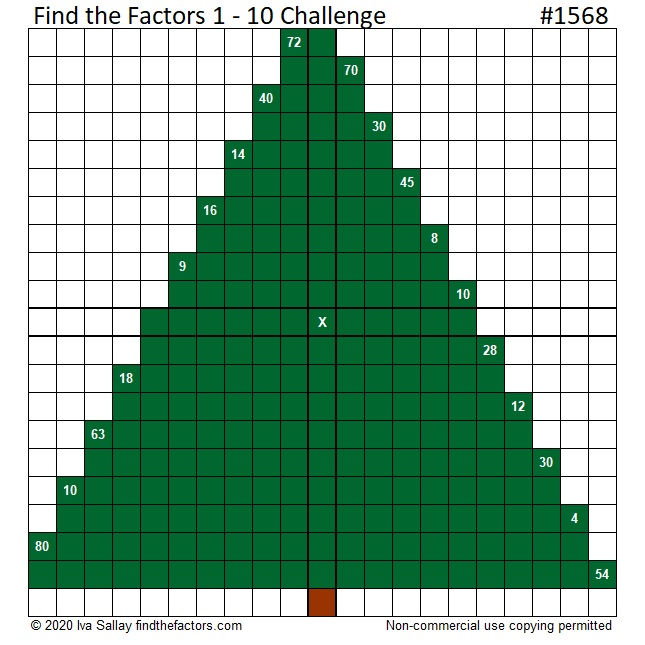Here is the same puzzle that won’t use so much ink to print:### 1568 Factor Tree:

Here is one of several possible factor trees for 1568:### Factors of 1568:

• 1568 is a composite number.
• Prime factorization: 1568 = 2 × 2 × 2 × 2 × 2 × 7 × 7, which can be written 1568 = 2⁵ × 7²
• 1568 has at least one exponent greater than 1 in its prime factorization so √1568 can be simplified. Taking the factor pair from the factor pair table below with the largest square number factor, we get √1568 = (√784)(√2) = 28√2
• The exponents in the prime factorization are 5 and 2. Adding one to each exponent and multiplying we get (5 + 1)(2 + 1) = 6 × 3 = 18. Therefore 1568 has exactly 18 factors.
• The factors of 1568 are outlined with their factor pair partners in the graphic below.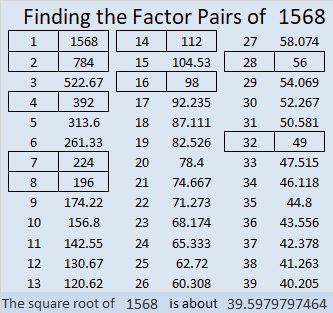### More about the Number 1568:

1568 is the difference of two squares SIX different ways:
393² – 391² = 1568,
198² – 194² = 1568,
102² – 94² = 1568,
63² – 49² = 1568,
57² – 41² = 1568, and
42² – 14² = 1568.